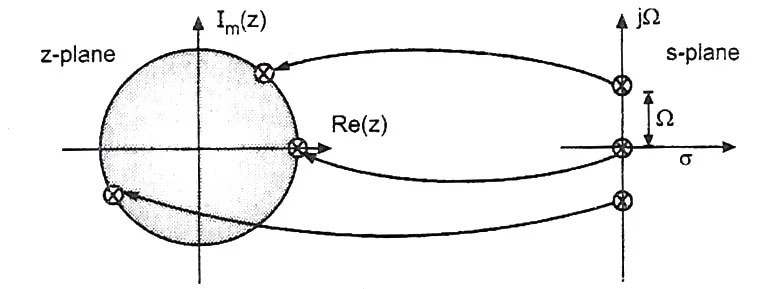View Course Path

# Relation of Z-transform with Fourier and Laplace transforms – DSP

In digital signal processing, we often have to convert a signal from its various representations. Interconversion between various domains like Laplace, Fourier, and Z is an important skill for any student. In this post, we will discuss the relationship between the three most common transformation methods. We will see the interconversion process both algebraically as well as graphically.

Contents

## Relationship between Laplace transform and Z-transform

We employ the Laplace transform in DSP in analyzing continuous-time systems. Conversely, the z-transform is used to analyze discrete-time systems. If you’d like to recap, here’s the difference between continuous-time systems and discrete-time systems.

The Laplace transform converts differential equations into algebraic equations. Whereas the Z-transform converts difference equations (discrete versions of differential equations) into algebraic equations.

The Laplace transform maps a continuous-time function f(t) to f(s) which is defined in the s-plane. In the s-plane, s is a complex variable defined as:$s\quad =\quad \sigma +j\Omega$

Similarly, the Z-transform maps a discrete time function f(n) to f(z) that is defined in the z-plane. Here z is a complex variable defined as:$z\quad =\quad r{ e }^{ j\omega }$

### Derivation

Consider a periodic train of impulses p(t) with a period T.$P(t)\quad =\quad \sum _{ n=-\infty }^{ \infty }{ \delta (t-nT) }$

Now consider a periodic continuous time signal x(nT).

Take a product of the above two signals as shown below.

Multiplying a continuous time signal with an impulse signal is known as the impulse sampling of a continuous time signal.${ x }_{ p }(t)\quad =\quad \sum _{ n=-\infty }^{ \infty }{ x(nT).\delta (t-nT) }$

Taking Laplace transform of the above signal and using the identity$L(\delta (t-nT))\quad =\quad { e }^{ -nsT }$

Thus,${ x }_{ p }(s)\quad =\quad \sum _{ n=-\infty }^{ \infty }{ x(nT).{ e }^{ -nsT } }$

Which can be written as:${ x }_{ p }(s)\quad =\quad \sum _{ n=-\infty }^{ \infty }{ x(nT).{ { (e }^{ st }) }^{ -n } }$

Compare this equation with that of z-transform$X(Z)\quad =\quad \sum _{ n=-\infty }^{ \infty }{ x(n).{ { z }^{ -n } } }$

Thus we finally get the relation:$z\quad =\quad { e }^{ sT }$ (Derived from the Impulse Invariant method)

Another representation:$s\quad =\quad \frac { 2(z-1) }{ T(z+1) }$ (Derived from Bilinear Transform method)

### Mapping the s-plane into the z-planeMapping of poles located at the imaginary axis of the s-plane onto the unit circle of the z-plane. This is an important condition for accurate transformation.Mapping of the stable poles on the left-hand side of the imaginary s-plane axis into the unit circle on the z-plane. Another important condition.Poles on the right-hand side of the imaginary axis of the s-plane lie outside the unit circle of the z-plane when mapped.

## Relationship between Fourier transform and Z-transform$X(\omega )\quad =\quad \sum _{ n=-\infty }^{ \infty }{ x(n).{ { e }^{ -j\omega n } } }$

And the equation for z-transform is$X(Z)\quad =\quad \sum _{ n=-\infty }^{ \infty }{ x(n).{ { z }^{ -n } } }$

Replacing the value of z in the above equation using$z\quad =\quad r{ e }^{ j\omega }$$X(Z)\quad =\quad \sum _{ n=-\infty }^{ \infty }{ x(n).{ { ({ re }^{ j\omega }) }^{ -n } } }$

Thus;$X(Z)\quad =\quad \sum _{ n=-\infty }^{ \infty }{ x(n){ { { r } }e^{ -j\omega n } } }$

For r = 1

That is, if we evaluate the above equation on the unit circle of the z-plane, we get:$X(Z)\quad =\quad \sum _{ n=-\infty }^{ \infty }{ x(n).{ { e }^{ -j\omega n } } }$

If you compare the above equation with the formula of the fourier transform, you can observe that the RHS of both the equations is the same. Thus we can say that the z-transform of a signal evaluated on a unit circle is equal to the fourier transform of that signal.

### Mapping between phase and frequency on the unit circle

In the z-plane,$z\quad =\quad r{ e }^{ j\omega }$, is a phasor with r being the magnitude and ω being the angle. This angle can be represented as:$\angle Z\quad =\quad { tan }^{ -1 }\left[ \frac { { I }_{ m }[z]\quad (Imaginary\quad axis\quad of\quad z-plane) }{ { R }_{ e }[z]\quad (Real\quad axis\quad of\quad z-plane) } \right]$

Or$\omega \quad =\quad { tan }^{ -1 }\left[ \frac { { I }_{ m }[z]\quad (Imaginary\quad axis\quad of\quad z-plane) }{ { R }_{ e }[z]\quad (Real\quad axis\quad of\quad z-plane) } \right]$

For a normal z-plane, this indicates that ω is going to vary from 0 to 2π. However, in this case, we have r=1 and we are trying to establish a relationship between FT and ZT. So we can say that since the fourier transform is equal to the z-transform, ω of the z-plane is equivalent to the frequencies of the fourier transform which by extension now vary from 0 to 2π too.

Now that you are comfortable with the interconversion between different domains, we can proceed to understand the peculiarities of each domain. If there is any query in your mind pertaining to the explanation above, let us know in the comments. We are always around to help.

This site uses Akismet to reduce spam. Learn how your comment data is processed.